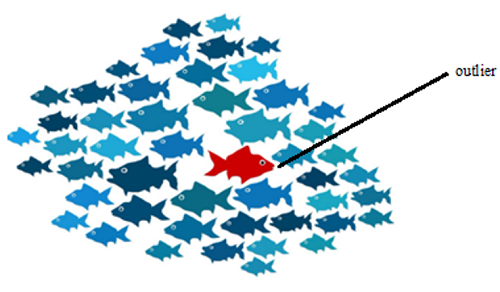# Data Mining Outlier Analysis: What It Is, Why It Is Used?

In this tutorial, we will learn about the outlier analysis in data mining and its related concepts like why outlier analysis, how outlier detection can improve business analysis, how to detect an outlier, common steps of algorithm, and, outlier analysis techniques. By Palkesh Jain Last updated : April 17, 2023

## Outlier Detection

Outlier detection in data mining seeks to identify trends in data that do not comply with expected behavior.Fig: An example of an outlier

## What are Outliers?

Outliers are a special concern in data analysis; it is most widely used in the identification of fraud, where outliers may demonstrate illegal conduct. Outlier Analysis is a technique that involves finding in the sample the anomalous observation. Outlier discovery and interpretation is also an interesting activity for data mining. An outlier is an aspect of a data set that stands out strongly from the rest of the results.

Outlier Analysis is an activity for data processing known as outlier mining. It has different application areas such as irregular use of credit cards or telecommunication systems, healthcare research to discover unusual reactions to medical procedures, and also to determine the advertisement expense nature of consumers.

## Why Outlier Analysis?

Most data mining techniques discard outlier's noise or anomalies, but the unusual incidents may be more interesting than the more frequently occurring in some applications such as fraud detection and hence the outlier analysis becomes important in such cases.

## How Outlier Detection Can Improve Business Analysis?

An organization should first think about whether they want to identify the outliers and what they can do with the information before evaluating the use of outlier analysis. To reveal the results they need to see and comprehend, this emphasis will help the organization to choose the correct form of analysis using diagrams or plotting. When an organization uses outlier analysis, it is necessary to validate the findings with an overall dataset.

## How to Detect an Outlier?

Clustering-based outlier identification using the nearest cluster distance. Each cluster has a mean value within the K-Means clustering technique. Objects belong to a cluster and are nearest to their mean value. First, we need to initialize the threshold value to define the Outlier in such a way that any distance of any data point greater than it from its nearest cluster marks it as an outlier for our intent. Then we need to find the mean distance between the test data and each cluster. Now, if the distance is greater than the threshold value between the test data and the nearest cluster to it, then the test data would be labelled as an outlier.

## Common Steps of Algorithm

• Initialize the value of the Threshold.
• Calculate the distance between the test data from the average of each cluster.
• Find the cluster closest to the test results
• If, then, (Distance > Threshold) Outlier
• Calculate each cluster's average.

## Outlier Analysis Techniques

The simplest method for outlier analysis is sorting. Load the dataset into a data processing method, such as a spreadsheet, and then arrange the values. Then, look at the spectrum of different data points. They can be viewed as outliers if some data points are substantially higher or lower than those in the dataset.

### Example

Let's take a look at an example of real sorting. Consider that a company's CEO gets a salary that is two times that of the other staff. They should look to ensure that no outliers are found in the dataset upon entering the data review process. They would be able to spot exceptionally high findings when sorting through the highest incomes. Knowing that the average pay is higher, a CEO salary analysis will stand out as an outlier.